Start typing, then use the up and down arrows to select an option from the list.
3:58 minutes
Problem 93
Textbook Question

# The following steps occur in the reaction of ethyl alcohol (CH3CH2OH) wiht oxygen to yield acetic acid (CH3CO2H). Show that equations 1 and 2 sum to give the net equation and calculate ΔH° for the net equation. (1) CH3CH2OH(l) + 1/2 O2(g) → CH3CHO (g) + H2O(l) ΔH° = -174.2 kJ (2) CH3CHO(g) + 1/2 O2(g) → CH3CO2H(l) ΔH° = -318.4 kJ (Net) CH3CH2OH(l) + O2(g) → CH3CO2H(l) + H2O(l) ΔH° = ?Verified Solution
This video solution was recommended by our tutors as helpful for the problem above.
70views

### Watch next

Master Hess's Law with a bite sized video explanation from Jules Bruno

Start learning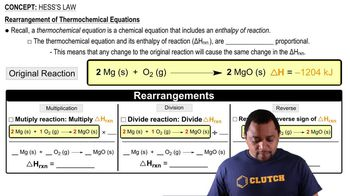03:11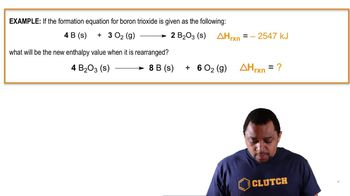01:39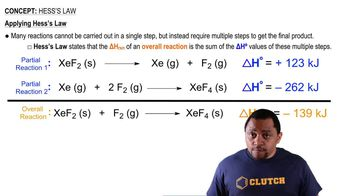02:03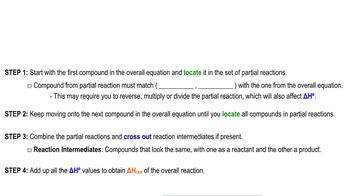05:43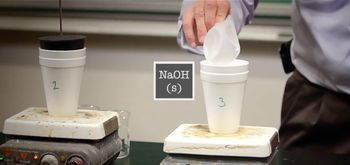07:46